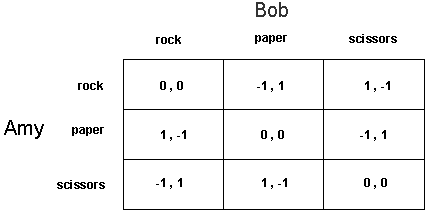Students / SubjectsHandbook > Game Theory > Game Theory Introduction - ContinuedPrinter Friendly

Game Theory Introduction

Example - Rock, Paper, and Scissors

Consider the ever popular game of "Rock, Paper and Scissors," which we all probably have played as kids. Two children play at once, so the list of participants is, say, Amy and Bob. The two simultaneously choose one of the three options; in this game, these are the strategies available to each, called: rock, paper, scissors. Now depepnding on what each of the two children chooses, the game is either won by one of the children or it is a draw. The rules of the game are as follows. If both choose the same option, the game is a draw. If one chooses rock and the other paper, paper wins. (Paper covers rock you know!) If one chooses rock and the other scissors, the rock wins. (Rock breaks scissors) If one chooses paper and the other scissors, scissors wins. (Scissors cut paper) Now let us consider the payoffs. Suppose that the payoff to a child for winning is a penny( +1), the payoff for losing is a penny (-1), and the payoff for a draw is 0. We can then represent the game with the following figure:Note the following:

• Since there are two players in this game, and each had three available strategies, the set of "strategy profiles" or "strategy arrays" forms a 3 X 3 table.

• In the above figure, Amy's strategies are listed as rows in the table, and Bob's strategies as columns.

• For each of the nine cells in the table, there is a pair of payoffs to the two players, first the payoff to Amy and then the payoff to Bob. So for example, if Amy chose to play "rock" (row 1) and Bob chose to play "scissors" (column 3), then Amy receives a payoff of +1 penny and Bob gets a payoff of -1.

How would you play?

Think of how Amy or Bob would play. If you had played this game before you would know that playing either rock or paper or scissors each time makes you predictable and vulnerable to your opponent. So if Amy played rock in all trials then all Bob has to do is to play paper. Similarly if Bob played paper in all trials then all Amy has to do to win all rounds is play scissors. So it seems that a good way to play this game is to be unpredictable in each trial, in the sense that you sometimes play rock and sometimes paper, and so forth.

The game theorists have a formal way of solving a game like this using the concept of ?mixed strategies.? For a formal discussion of mixed strategy click here.

Continue